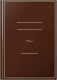# Probability and Random ProcessesWiley, Nov 4, 2005 - Mathematics - 420 pages
A resource for probability AND random processes, with hundreds of worked examples and probability and Fourier transform tables

This survival guide in probability and random processes eliminates the need to pore through several resources to find a certain formula or table. It offers a compendium of most distribution functions used by communication engineers, queuing theory specialists, signal processing engineers, biomedical engineers, physicists, and students.

Key topics covered include:
* Random variables and most of their frequently used discrete and continuous probability distribution functions
* Moments, transformations, and convergences of random variables
* Characteristic, generating, and moment-generating functions
* Computer generation of random variates
* Estimation theory and the associated orthogonality principle
* Linear vector spaces and matrix theory with vector and matrix differentiation concepts
* Vector random variables
* Random processes and stationarity concepts
* Extensive classification of random processes
* Random processes through linear systems and the associated Wiener and Kalman filters
* Application of probability in single photon emission tomography (SPECT)

More than 400 figures drawn to scale assist readers in understanding and applying theory. Many of these figures accompany the more than 300 examples given to help readers visualize how to solve the problem at hand. In many instances, worked examples are solved with more than one approach to illustrate how different probability methodologies can work for the same problem.

Several probability tables with accuracy up to nine decimal places are provided in the appendices for quick reference. A special feature is the graphical presentation of the commonly occurring Fourier transforms, where both time and frequency functions are drawn to scale.

This book is of particular value to undergraduate and graduate students in electrical, computer, and civil engineering, as well as students in physics and applied mathematics. Engineers, computer scientists, biostatisticians, and researchers in communications will also benefit from having a single resource to address most issues in probability and random processes.

### What people are saying -Write a review

We haven't found any reviews in the usual places.